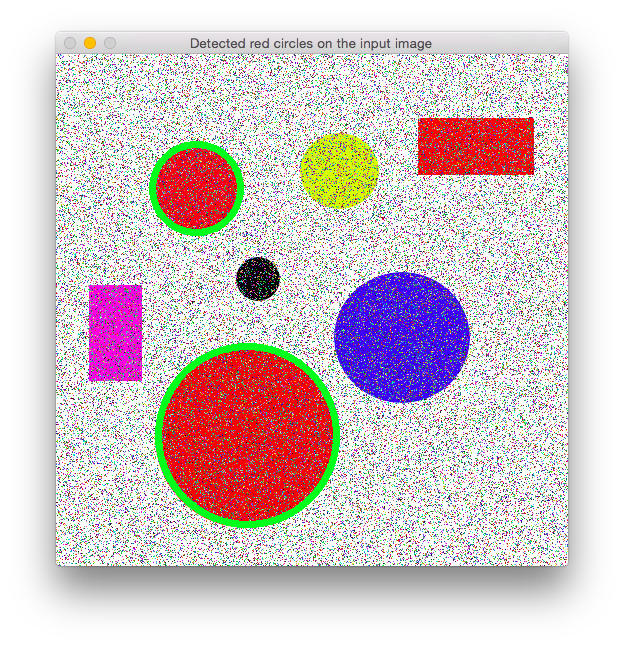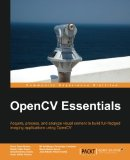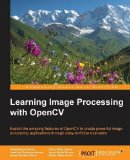# Detect red circles in an image using OpenCV

## Posted on May 8, 2015 by Paul

The code for this post is on GitHub: https://github.com/sol-prog/OpenCV-red-circle-detection.

A few days ago someone asked me, in an email, if it is possible to detect all red circles in an image that contains circles and rectangles of various colors. I thought this problem could be of certain interest to the readers of this blog, hence the present article.

From the many possible approaches to the problem of red circles detection, two seem straightforward:

• Detect all circles from the input image and keep only the ones that are filled with red.
• Threshold the input image in order to keep only the red pixels, search for circles in the result.

I found the second approach to be slightly better than the first one (less false positives), so I am going to present it in this post.

I will use the OpenCV library and C++, but you can easily follow along with any of the other OpenCV bindings (C, Python, Java).

Lets start by thresholding the input image for anything that is not red. Instead of the usual RGB color space we are going to use the HSV space, which has the desirable property that allows us to identify a particular color using a single value, the hue, instead of three values. As a side note, in OpenCV H has values from 0 to 180, S and V from 0 to 255. The red color, in OpenCV, has the hue values approximately in the range of 0 to 10 and 160 to 180.

Next piece of code converts a color image from BGR (internally, OpenCV stores a color image in the BGR format rather than RGB) to HSV and thresholds the HSV image for anything that is not red:

Take the next input image as an example: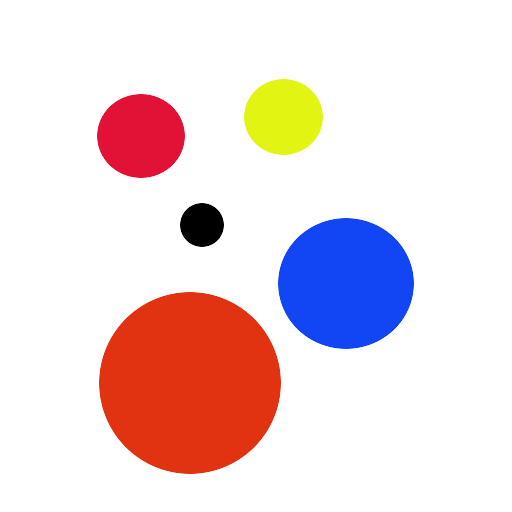if we use the above piece of code, this is what we get: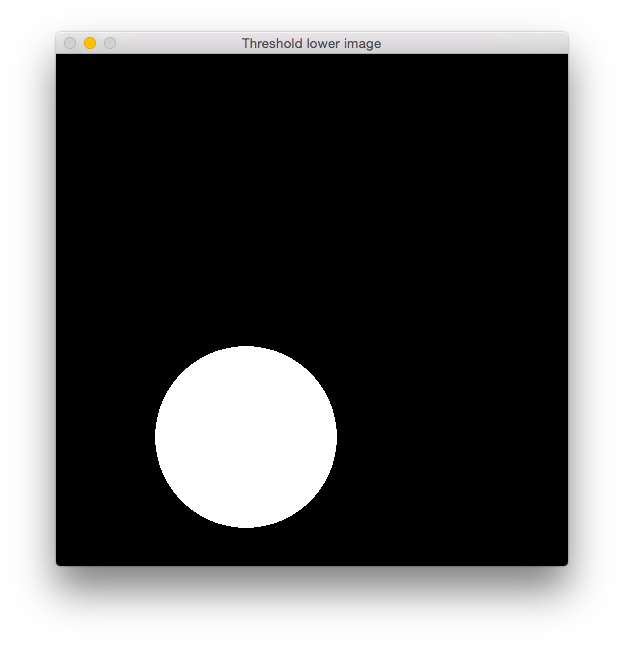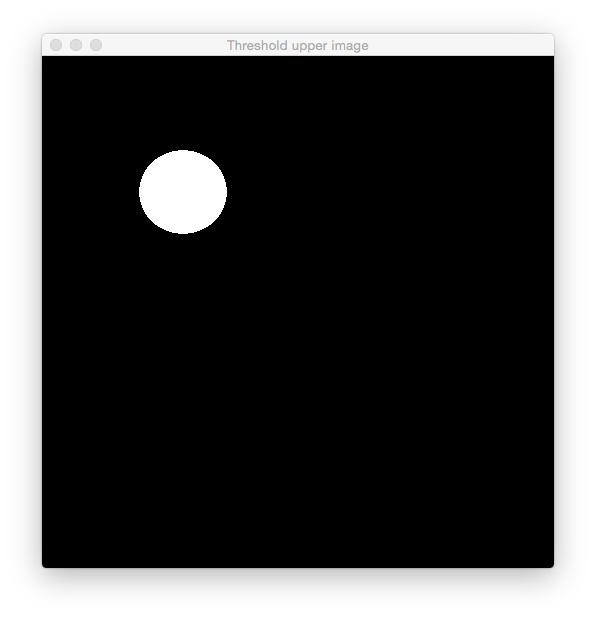As you can see, the first threshold image captured the big red circle from the input image, while the second threshold image has captured the smaller red circle. Typically, you won’t see such a clear separation between the two red ranges. I’ve slightly cheated when I filled the circles in GIMP and used hue values from both intervals, in order to show you that a similar situation can arrive in practice.

Next step is to combine the above threshold images and slightly blur the result, in order to avoid false positives: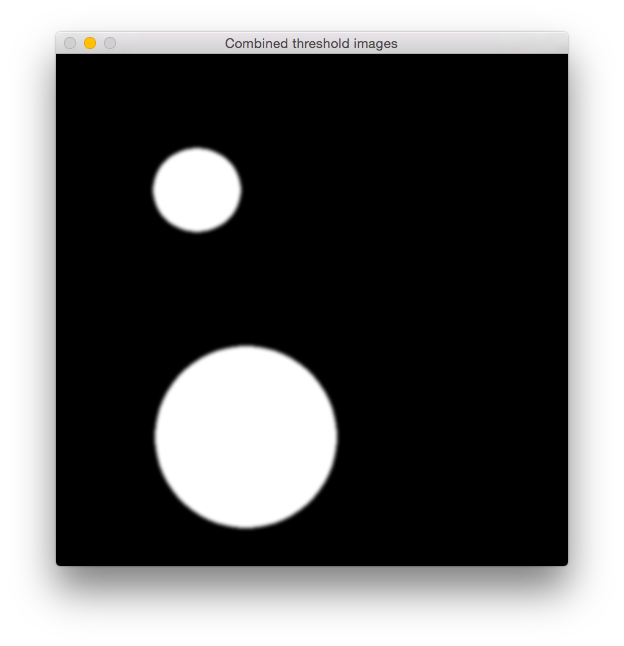Once we have the threshold image that contains only the red pixels from the original image, we can use the circle Hough Transform to detect the circles. In OpenCV this is implemented as HoughCircles:

As a side note, parameters 6 and 7 from the HoughCircles must be usually tuned from case to case in order to detect circles. All found circles are stored in the circles vector from the above piece of code, using this information we can outline the detected circles on the original image: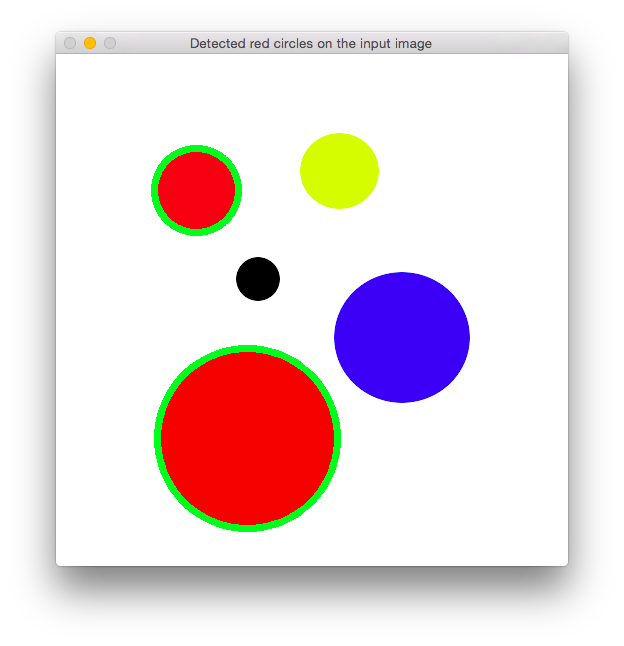Lets try the code on a slightly more complex image: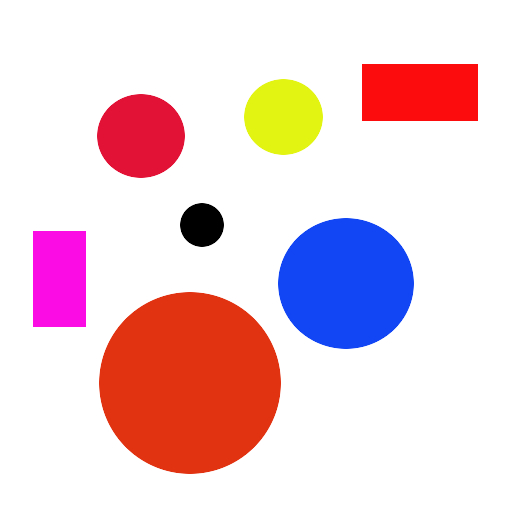and the result: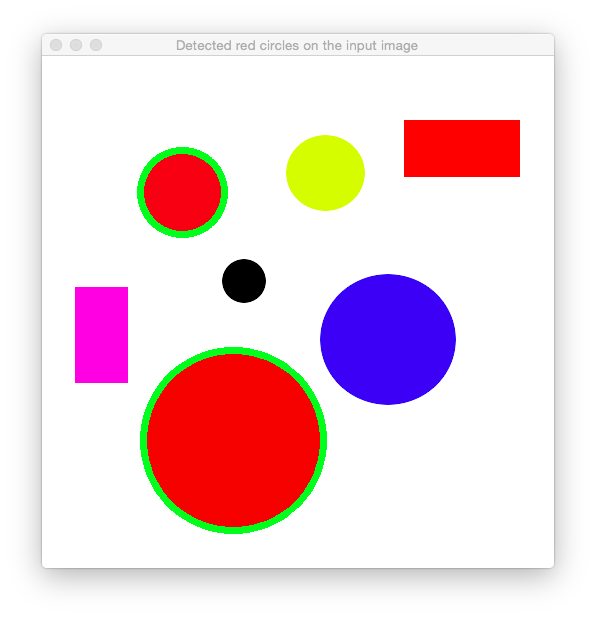Adding some noise to the same input image as above: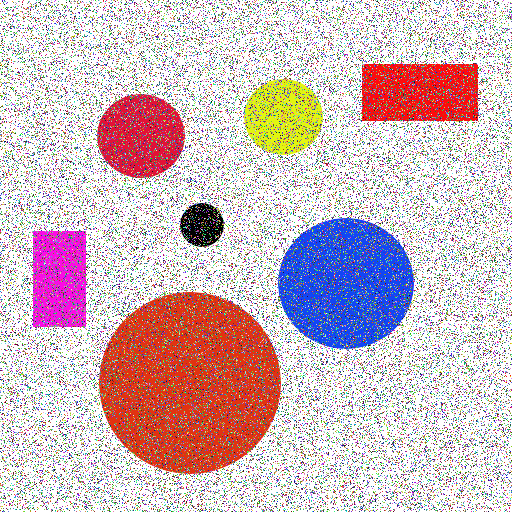and the incredible result: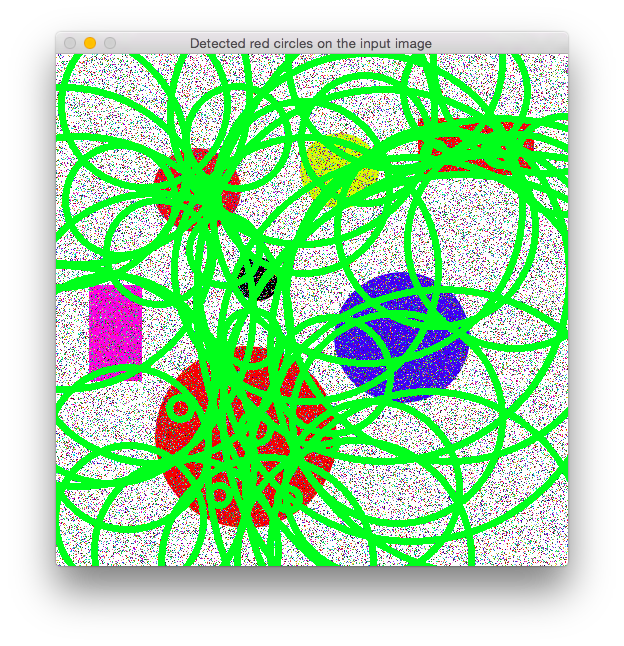Ouch! Apparently the noise from the input image fooled the Hough detector and now we have more circles than we’ve expected. A simple cure is to filter the input image before the BGR to HSV conversion, for this kind of noise usually a median filter works best:

and now the result is much improved: